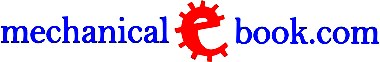Custom Search
 Mechanical Definitions Tariffs : The rate at which electrical energy is supplied to a consumer. Simple Tariff : It is a fixed rate per unit of energy consumed. It can be termed as Uniform Rate Tariff. Flat Rate Tariff : Under this, different types of consumers as domestic, small scale industrials, large industries, bulkpower consumers, etc. are charged at different uniform rate per kWh of electric energy. Block Rate Tariff : The tariff is called block rate tariff when a given block of energy is charged at a specific rate and the succeeding block of energy are charged at progressively reduced rates. Two Part Tariff : In this case, charges are made on the basis of maximum demand of consumer and the units consumed. Generally applied to industrial consumers who have appreciable maximum demand. Total Charges = Rs (b X kW + c X kWh) where b = charge per kW of maximum demand and c = charge per kWh of energy consumed Power Factor Tariff : In this case, power factor of the consumer is taken into account. Three Part Tariff : As the cost of power generation can be divided into three parts such as fixed charges, semi-fixed charges and operating charges, proportionately three part tariff will consist of Total Charges = Rs (a + bx X kW + c X kWh) where a = fixed charge made during the billing period, b = charges per kW of maximum demand, c = charge per kWh of energy consumed. Thermodynamic Cycle : It is a series of thermodynamic processes which returns a system to its initial state. In other words, it consists of a series of operations which take place in a certain order and restore the initial condition. Thermodynamics : It deals with relations between heat and work. It predicts many relationships between properties of matter. Thermodynamics System : It is defined as any prescribed and identifiable collection of matter upon which attention is focused for investigations. Turbomachine : It is used to convert energy in fluids into mechanical energy such as, steam, gas or water turbines. It is also used for converting mechanical energy into energy of fluids.